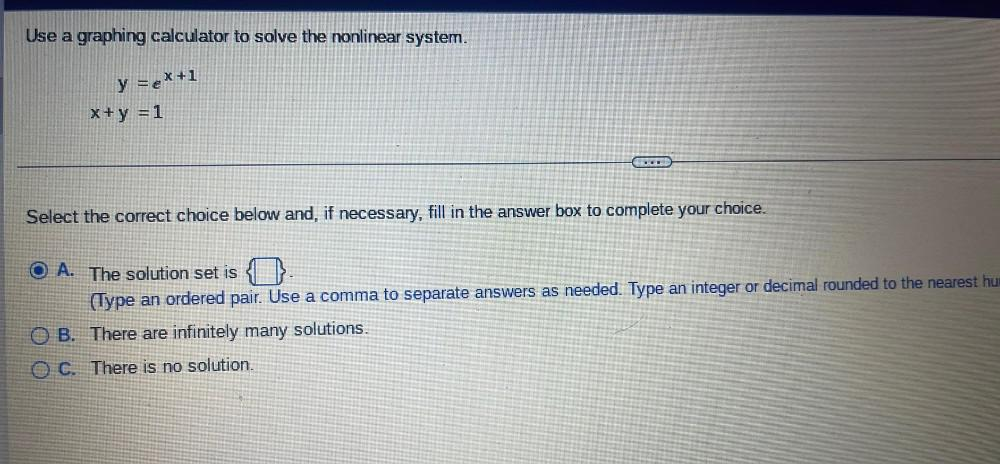Question:

# Use a graphing calculator to solve the nonlinear system. y =e^(X+1) X+y =1 Select the correct choice below and, if necessary, fill in the answer box to complete your choice. A). The solution set is (Use a graphing calculator to solve the nonlinear system. y =e^(X+1) X+y =1 Select the correct choice below and, if necessary, fill in the answer box to complete your choice. A). The solution set is (Type an ordered pair. Use a comma to separate answers as needed. Type an integer or decimal rounded to the nearest hundred) B). There are infinitely many solutions. C). There is no solution.